JEE  >  Test: Sets- 1

# Test: Sets- 1

Test Description

## 25 Questions MCQ Test Mathematics (Maths) Class 11 | Test: Sets- 1

Test: Sets- 1 for JEE 2023 is part of Mathematics (Maths) Class 11 preparation. The Test: Sets- 1 questions and answers have been prepared according to the JEE exam syllabus.The Test: Sets- 1 MCQs are made for JEE 2023 Exam. Find important definitions, questions, notes, meanings, examples, exercises, MCQs and online tests for Test: Sets- 1 below.
Solutions of Test: Sets- 1 questions in English are available as part of our Mathematics (Maths) Class 11 for JEE & Test: Sets- 1 solutions in Hindi for Mathematics (Maths) Class 11 course. Download more important topics, notes, lectures and mock test series for JEE Exam by signing up for free. Attempt Test: Sets- 1 | 25 questions in 25 minutes | Mock test for JEE preparation | Free important questions MCQ to study Mathematics (Maths) Class 11 for JEE Exam | Download free PDF with solutions
 1 Crore+ students have signed up on EduRev. Have you?
Test: Sets- 1 - Question 1

### Which of the following is the empty set?

Detailed Solution for Test: Sets- 1 - Question 1

As we know that the set of real numbers is made by combining the set of rational numbers and the set of irrational numbers.
The real numbers include natural numbers or counting numbers, whole numbers, integers, rational numbers(fractions and repeating or terminating decimals), and irrational numbers.

So, now as we can see that the set given in all the above options have real numbers. But there is one additional condition given in each set which defines the elements of the set.

So, we had to solve that additional condition to find the elements of the set.

Option A: x2 – 1 = 0
Adding 1 to both sides of the above equation.
x = 1
Taking square root to both sides of the above equation.
x = 1, -1
So, the set in option A will be {1, -1} because 1 and -1 are also real numbers.
Hence, option A is not correct because this set is not empty.

Option B: x2 + 1 = 0
Subtracting 1 to both sides of the above equation.
x2  = -1
Taking square root to both sides of the above equation.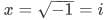So, the set in option B will have no elements because i (iota) is not a real number, it is a complex number.

Since there cannot be more than one option correct. So, we need not to check all options.

Hence, option B is correct because this set is an empty set.

Test: Sets- 1 - Question 2

### What is the cardinality of the set of odd positive integers less than 10?

Detailed Solution for Test: Sets- 1 - Question 2
• Set S of odd positive integer less than 10 is {1, 3, 5, 7, 9}.
• Then, the cardinality of set S, i.e., the number of elements in the set, is 5.
Test: Sets- 1 - Question 3

### Consider the following relations: (i) A - B = A - (A ∩ B) (ii) A = (A ∩ B) ∪ (A - B) (iii) A - (B ∪ C) = (A - B) ∪ (A - C) Which of these is/are correct?

Detailed Solution for Test: Sets- 1 - Question 3

Using the Venn Diagram,
(i) A - B = A - (A ∩ B) is true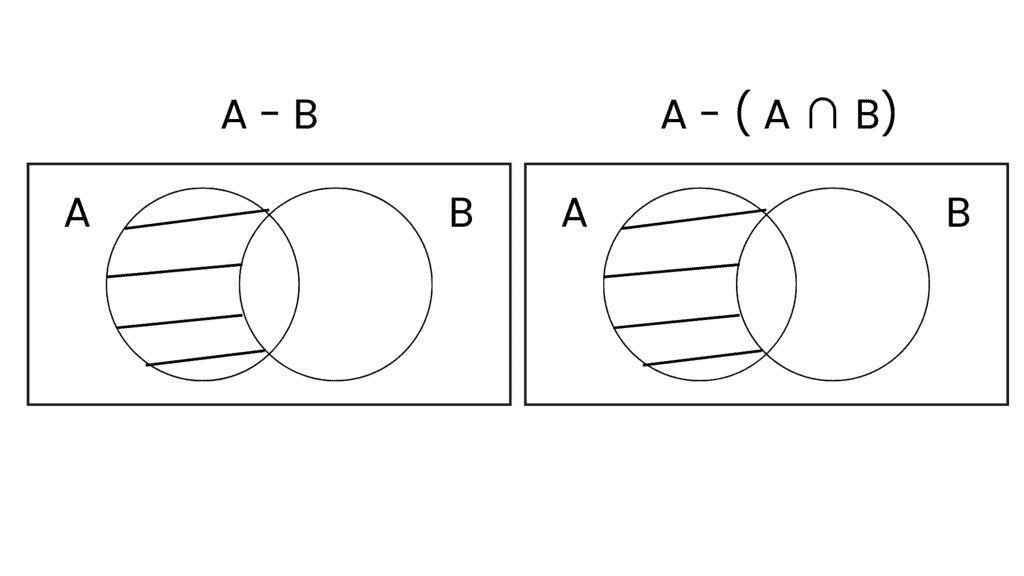(ii) A = (A ∩ B) ∪ (A - B) is true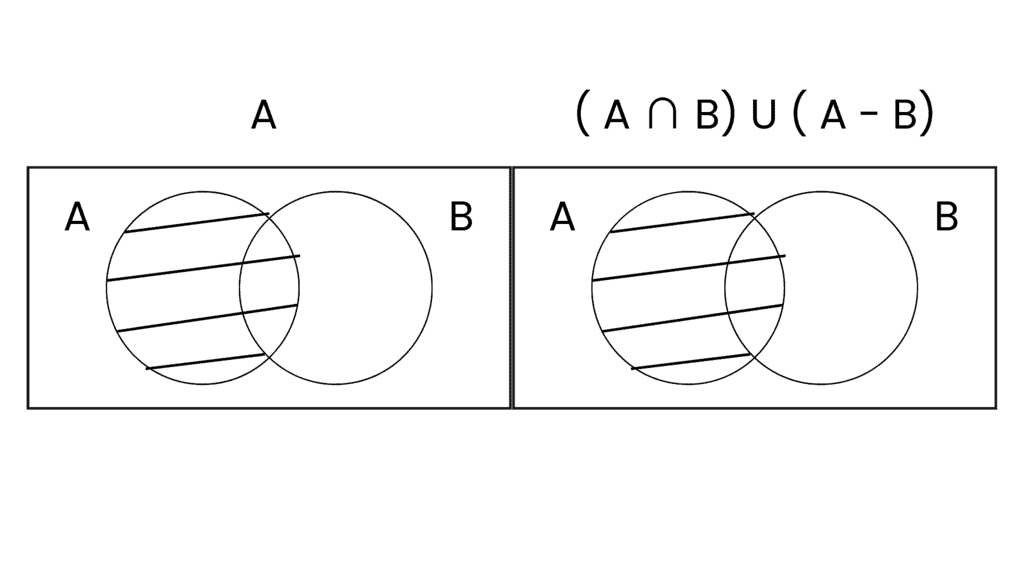(iii) A - (B ∪ C) = (A - B) ∪ (A - C) is false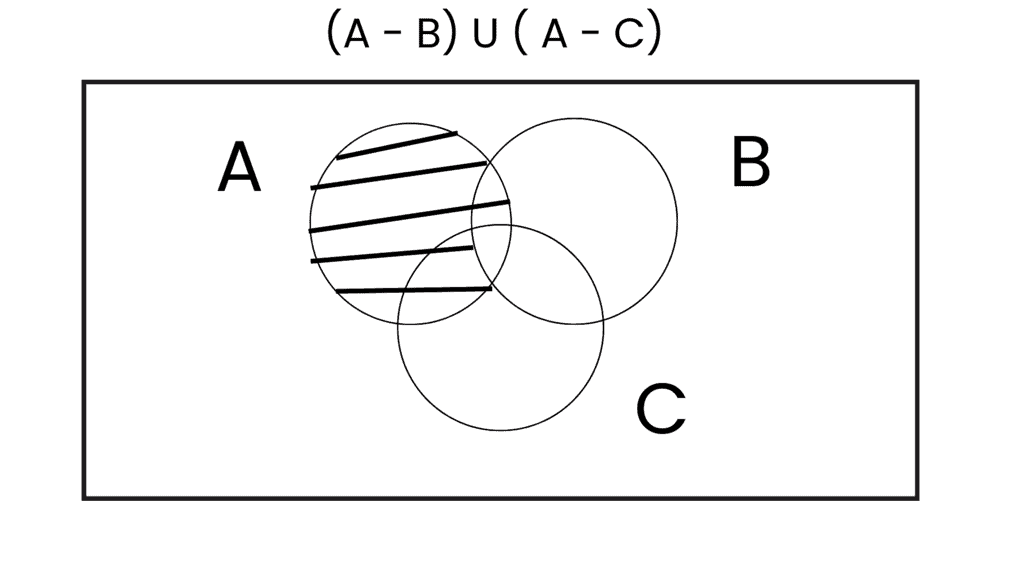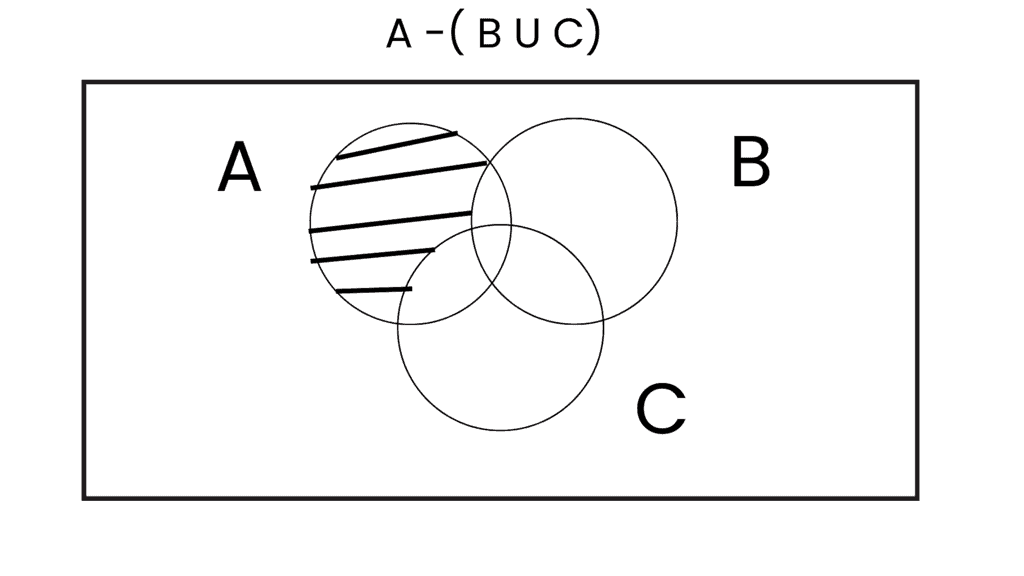Test: Sets- 1 - Question 4

If A and B be any two sets, then (A ∪ B)' is equal to:

Detailed Solution for Test: Sets- 1 - Question 4

By applying De Morgan's Law,
(A U B)' = A' ∩ B'

Test: Sets- 1 - Question 5

The smallest set A such that A ∪ {1, 2} = {1, 2, 3, 5, 9} is:

Detailed Solution for Test: Sets- 1 - Question 5

A ∪ {1, 2} = {1, 2, 3, 5, 9}
If A = {3, 5, 9}
Then, A ∪ {1, 2} = {1, 2, 3, 5, 9}
So, the minimum set of A = {3, 5, 9}

Test: Sets- 1 - Question 6

In a city, 20 per cent of the population travels by car, 50 per cent travels by bus and 10 per cent travels by both car and bus. Then persons travelling by car or bus is:

Detailed Solution for Test: Sets- 1 - Question 6

► n(Persons travelling by car) = 20 = n(C)
► n(Persons travelling by bus) = 50 = n(B)
► n(Persons travelling by both car and bus) = 10 = n(C ∩ B)
∴ n(C ∪ B) = n(C) + n(B) - n(C ∩ B)
= 20 + 50 - 10
= 60

Test: Sets- 1 - Question 7

Let U = {1, 2, 3, 4, 5, 6, 7, 8, 9, 10}, A = {1, 2, 5}, B = {6, 7}. Then A ∩ B’ is:

Detailed Solution for Test: Sets- 1 - Question 7
• B' gives us all the elements in U other than 6 and 7 i.e., B' = {1, 2, 3, 4, 5, 8, 9, 10}
• The intersection of this set with A will be the common elements in both of these (A and B') i.e., = {1, 2, 5} which is set A itself.
Test: Sets- 1 - Question 8

In the rule method, the null set is represented by:

Detailed Solution for Test: Sets- 1 - Question 8
• This method involves specifying a rule or condition which can be used to decide whether an object can belong to the set.
• This rule is written inside a pair of curly braces and can be written either as a statement or expressed symbolically or written using a combination of statements and symbols.
• {x :  x ≠ x}
This implies a null set.
Test: Sets- 1 - Question 9

If A and B are two sets, then A ∪ B = A ∩ B if:

Detailed Solution for Test: Sets- 1 - Question 9

If A ∪ B = A ∩ B then A = B.
Example: Let A = {1, 2, 3, 4} & B = {1, 2, 3, 4}
(AUB) = (A⋂B)
⇒{1,2,3,4} = {1,2,3,4}

Test: Sets- 1 - Question 10

If  A = {2, 3, 4, 8, 10}, B = {3, 4, 5, 10, 12}, C = {4, 5, 6, 12, 14} then (A ∩ B) ∪ (A ∩ C) is equal to:

Detailed Solution for Test: Sets- 1 - Question 10

A = {2, 3, 4, 8, 10}
B = {3, 4, 5, 10, 12}
C = {4, 5, 6, 12, 14}
► (A ∩ B) = {3,4,10}
► (A ∩ C) = {4}
► (A ∩ B) U (A ∩ C) = {3,4,10}

Test: Sets- 1 - Question 11

The shaded region in the given figure is: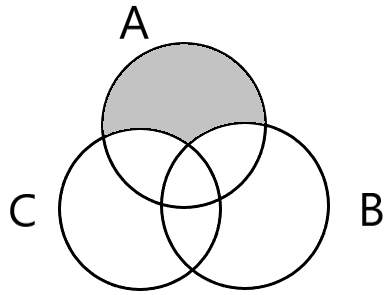Detailed Solution for Test: Sets- 1 - Question 11
• (A - B) is A but not B which means A excluding the intersection of A and B.
• Similarly, in the figure, the shaded region is A but not C and B which means A excluding B and C. But if B and C both are excluded from A then the intersection of A, B and C get excluded two times.
• So, we take B ∪ C to be excluded from A. Therefore the answer is A - (B ∪ C).
Test: Sets- 1 - Question 12

Let A and B be two sets containing four and two elements respectively.Then the number of subsets of the set A × B,each having atleast three elements is:

Detailed Solution for Test: Sets- 1 - Question 12

⇒ Number of elements in Set A = 4
⇒ Number of elements in Set B = 2
∴ Number of elements in set (A × B) = 8
∴ Total number of subsets of (A×B) = 28 = 256
⇒ Number of subsets having 0 elements = 8C0 = 1
⇒ Number of subsets having 1 element = 8C1 = 8
⇒ Number of subsets having 2 elements = 8C2 = 28
∴ Number of subsets having atleast 3 elements = 256 - 1 - 8 - 28 = 219

Test: Sets- 1 - Question 13

Let A and B be two sets then (A ∪ B)′ ∪ (A′ ∩ B) is equal to:

Detailed Solution for Test: Sets- 1 - Question 13

From Venn-Euler's Diagram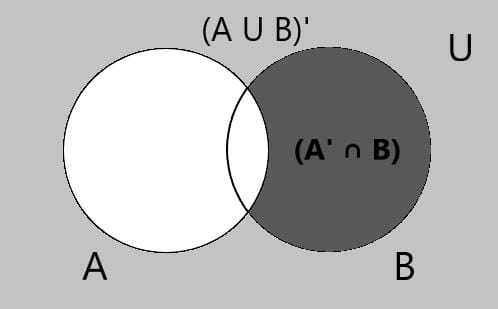∴ (A ∪ B)′ ∪ (A′ ∩ B) = A′

Test: Sets- 1 - Question 14

The number of elements in the set {(ab) : 2a2 + 3b2 = 35, a∈ Z}, where Z is the set of all integers, is:

Detailed Solution for Test: Sets- 1 - Question 14

2a2 + 3b2 = 35

3b2 = 35 – 2a2

Maximum value on RHS is 35.

3b2 ≤ 35

b2 ≤ 11.66

b = 0, ± 1, ± 2, ± 3….

3b2 = 35 – 2a2

Any number multiplied by 2 give even number.

3b2 is odd.

If b = 0, then 3b2 is not odd.

If b = ± 1, then 3bis odd.

If b = ± 2, then 3bis even.

If b = ± 3, then 3bis odd.

3b2 = 35 – 2a2

If b = ± 1, then b2 = 1,

3 = 35 – 2a2

2a2 = 32

a2 = 16

a = ± 4

If b = ± 3, then b2 = 9

27 = 35 – 2a2

2a2 = 8

a2 = 4

a = ± 2

[(4, 1), (4, -1), (-4, 1), (-4, -1), (2, -3), (2, 3), (-2, 3), (-2, -3)]

Therefore there are 8 elements in total.

Test: Sets- 1 - Question 15

Given n(U) = 20, n(A) = 12, n(B) = 9, n(A ∩ B) = 4, where U is the universal set, A and B are subsets of U, then n((A ∪ B)c) =

Detailed Solution for Test: Sets- 1 - Question 15
• n(A ∪ B) = n(A) + n(B) − n(A ∩ B) = 12 + 9 − 4 = 17
• Now, n((A ∪ B)c) = n(U) − n(A ∪ B) = 20 − 17 = 3
Test: Sets- 1 - Question 16

In a certain town, 25% families own a cell phone,15% families own a scooter and 65% families own neither a cell phone nor a scooter. If 1500 families own both a cell phone and a scooter, then the total number of families in the town is:

Detailed Solution for Test: Sets- 1 - Question 16

Step-by-step Explanation:
Since we have given that,
Percentage of families own a cell phone = 25%
Percentage of families own a scooter = 15%
Percentage of families neither own = 65%
So, it becomes,
P(C ∪ S)= 1 - P(C ∪ S)
0.65 = 1 - P(C ∪ S)
1 - 0.65 = P(C ∪ S)
P(C ∪ S) = 0.35
So, it becomes,
P(C ∪ S) = P(C) + P(S) - P(C ∩ S)
0.35 = 0.25 + 0.15 - x
0.35 = 0.40 - x
x = 0.05
Thus, 0.05 x Total number of families in town = 1500
Total number of families in the town =
1500 / 0.05 = 30,000
Hence, the total number of families in the town is 30,000.

Test: Sets- 1 - Question 17

If A = {2, 3, 4, 8, 10}, B = {3, 4, 5, 10, 12} and C = {4, 5, 6, 12, 14}, then (A ∪ B) ∩ (A ∪ C) is equal to:

Detailed Solution for Test: Sets- 1 - Question 17

A = {2,3,4,8,10}, B = {3,4,5,10,12} and C = {4,5,6,12,14}
⇒ (AUB) = {2,3,4,5,8,10,12}
⇒ (AUC) = {2,3,4,5,6,8,10,12,14}
⇒ (AUB) ⋂ (AUC) = {2,3,4,5,8,10,12}

Test: Sets- 1 - Question 18

If A = {2, 4, 5}, B = {7, 8, 9}, then n(A × B) is equal to:

Detailed Solution for Test: Sets- 1 - Question 18

n(A × B) = n(A).n(B)
⇒ 3 × 3 = 9
A × B = {(2, 7), (2, 8), (2, 9), (4, 7), (4,8), (4, 9), (5, 7), (5, 8), (5, 9)}

Test: Sets- 1 - Question 19

If A and B are disjoint, then n (A ∪ B) is equal to:

Detailed Solution for Test: Sets- 1 - Question 19

As n(A ∪ B) = n(A) + n(B) - n(A ∩ B)
But in case of disjoint sets, n(A ∩ B) = 0
∴ n(A ∪ B) = n(A) + n(B)

Test: Sets- 1 - Question 20

The number of non-empty subsets of the set {1, 2, 3, 4} is:

Detailed Solution for Test: Sets- 1 - Question 20

The number of non-empty subsets = 2n − 1 = 24 − 1 = 16 − 1 = 15
The subsets are:
[{1}, {2}, {3}, {4}, {1,2}, {1,3}, {1,4}, {2,3}, {2,4}, {3,4}, {1,2,4}, {1,2,3}, {1,3,4} ,{2,3,4}, {1,2,3,4}]

Test: Sets- 1 - Question 21

The set of intelligent students in a class is:

Detailed Solution for Test: Sets- 1 - Question 21

As the opinions of different person is different about the intelligent student. So, we can't exactly have the same student's name in a set, hence it is not a well-defined collection.

Test: Sets- 1 - Question 22

If A and B are two sets, then A ∩ (B − A) is:

Detailed Solution for Test: Sets- 1 - Question 22

A ∩ (B − A) = ψ,[∵ x ∈ B − A ⇒ x∉ A]

Test: Sets- 1 - Question 23

If n(A) = 3, n(B) = 6 and A ⊆ B. Then the number of elements in A ∪ B is equal to:

Detailed Solution for Test: Sets- 1 - Question 23

Since A ⊆ B
∴ A ∪ B = B
So, n(A ∪ B) = n(B) = 6

Test: Sets- 1 - Question 24

In a class of 100 students, 55 students have passed in Mathematics and 67 students have passed in Physics. Then the number of students who have passed in Physics only is:

Detailed Solution for Test: Sets- 1 - Question 24
• Let M denote the sets of students who have passed in Mathematics and P denote the sets of students who have passed in Physics.
• Thus from the question we get:
► n(M∪P) = 100
► n(M) = 55
► n(P) = 67
• n(M∩P) = n(M) + n(P) - n(M∪P)
► n(M∩P) = 55 + 67 - 100
► n(M∩P) = 22
• ∴ The no. of who passed in physics only is given by:
= n(P) - n(M∩P) = 67 - 22 = 45
Test: Sets- 1 - Question 25

If A = {1, 2, 3, 4}, B = {4, 5, 6, 7}, then A - B = ?

Detailed Solution for Test: Sets- 1 - Question 25
• (A - B) is nothing but the elements which are present in set A but not present in set B.
• In other words, it is elements of set A excluding the elements which are in set B.
• Here A = {1, 2, 3, 4} and B = {4, 5, 6, 7}
So, (A - B) = {1, 2, 3}

## Mathematics (Maths) Class 11

156 videos|176 docs|132 tests
 Use Code STAYHOME200 and get INR 200 additional OFF Use Coupon Code
Information about Test: Sets- 1 Page
In this test you can find the Exam questions for Test: Sets- 1 solved & explained in the simplest way possible. Besides giving Questions and answers for Test: Sets- 1, EduRev gives you an ample number of Online tests for practice

## Mathematics (Maths) Class 11

156 videos|176 docs|132 tests